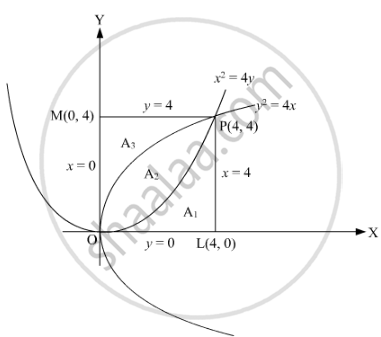Share

# Prove that the curves y^2 = 4x and x^2 = 4y divide the area of square bounded by x = 0, x = 4, y = 4 and y = 0 into three equal parts. - CBSE (Science) Class 12 - Mathematics

ConceptArea of the Region Bounded by a Curve and a Line

#### Question

Prove that the curves y2 = 4x and x2 = 4y divide the area of square bounded by x = 0, x = 4, y = 4 and y = 0 into three equal parts.

#### SolutionA1, A2, Aare the areas denoted in the figure. We need to prove A1 = A= A3.

A_1 = ∫_0^4y1dx

= ∫_0^4x^2/4dx

=1/4[x^3/3]_0^4

=16/3 sq. units

A_2=∫_0^4(y_2−y_1)dx

=∫_0^4(sqrt(4x)−x^2/4)dx

= [4/3x^3/2−x^3/12]_0^4

=16/3sq. units

A_3=area bounded by y^2=4x, y=0 and y=4

=∫_0^4x_1dy=∫_0^4y^2/4dy=1/4[y^3/3]_0^4=16/3

therefore A_1=A_2 =A_3 =16/3 sq. units

Thus, y2 = 4x and x2 = 4y divide the area of square bounded by x = 0, x = 4, y = 4 and y = 0 into three equal parts.

Is there an error in this question or solution?

#### Video TutorialsVIEW ALL 

Solution Prove that the curves y^2 = 4x and x^2 = 4y divide the area of square bounded by x = 0, x = 4, y = 4 and y = 0 into three equal parts. Concept: Area of the Region Bounded by a Curve and a Line.
S